UPSC  >  Speed, Time & Distance: Solved Examples- 3

# Speed, Time & Distance: Solved Examples- 3 - CSAT Preparation - UPSC

Question 16: Ramesh takes 6.5 hours to go from city A to city B at 3 different speeds 30 kmph, 45 kmph, and 60 kmph covering the same distance with each speed. The respective mileages per liter of fuel are 11 km, 14 km and 18 km for the above speeds. Ramesh's friend Arun is an efficient driver and wants to minimise his friend's car's fuel consumption. So he decides to drive Ramesh's car one day from city A to city B. How much fuel will he be able to save?
A. 4.2 liters
B. 4.5 liters
C. 0.7 liters
D. 0.3 liters
Option: B
Explanation:
Total time = 6.5 hrs
Let the total distance be x
Ramesh's speeds = 30, 45, 60 kmph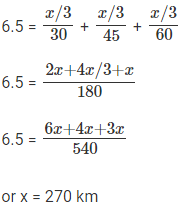or x = 270 km
With each speed distance covered = 270/3 = 90 km
Fuel consumed when driving at 30 = 90/11 = 8.1 l
Fuel consumed when driving at 45 = 90/14 = 6.4 l
Fuel consumed when driving at 60 = 90/18 = 5
Total fuel = 8.1 + 6.4 + 5 = 19.5 liters
When Arun drives the car, he should drive at 60 kmph throughout so that he gets the maximum mileage!
Fuel consumption when driving at 60 for entire journey = 270/18 = 15 liters
The question is " So he decides to drive Ramesh's car one day from city A to city B. How
much fuel will he be able to save? "
Difference = 19.5 – 15 = 4.5
Hence, the answer is 4.5 liters.

Question 17: Amar, Akbar and Antony decide to have a 'x' m race. Antony completes the race 14 m ahead of Amar. Akbar finishes 20 m ahead of Antony and 32 m ahead of Amar. What is Amar’s speed?
A. 9/10th of Antony's speed
B. 5/8th of Akbar's speed
C. 14/15th of Antony's speed
D. 10/7th of Akbar's speed
Option: A
Explanation:
When Antony completes, Amar would have run (x – 14) m; Time taken is the same
Ratio of Amar’s speed : Antony’s speed =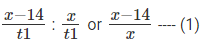When Akbar finishes, Amar would have run (x – 32) m; Antony would have run (x – 20) m; Time taken is the same At this point, the ratio of Amar’s speed : Antony’s speed =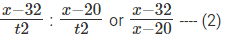Equating (1) and (2), we get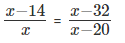x2 – 34x + 280 = x2 – 32x
2x = 280
Or x = 140 m
Ratio of Amar’s speed : Antony’s speed = (140−14)/140  = 126/140 = 910
The question is "What is Amar’s speed?"
Hence, Amar's Speed is 9/10th of Antony's Speed

Question 18: Tom, Jerry and Bill start from point A at the same time in their cars to go to B. Tom reaches point B first and turns back and meets Jerry at a distance of 9 miles from B. When Jerry reaches B, he too turns back and meets Bill at a distance of 7 miles from B. If 3 times the speed with which Tom drives his car is equal to 5 times Bill’s speed, what could be the distance between the points A and B?
A. 40 miles
B. 24 miles
C. 31 miles
D. 63 miles
Option: D
Explanation:
Let the distance between A and B be ‘x’ miles.
Let their speeds be T, J and B in miles/hour
When Tom meets Jerry:
Distance travelled by Tom = x + 9
Distance travelled by Jerry = x – 9
Time taken will be the same =>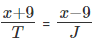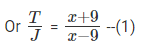When Jerry meets Bill:
Distance travelled by Jerry = x + 7
Distance travelled by Bill = x – 7
Time taken will be the same =>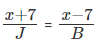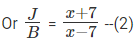Given 3T = 5B
or T/B = 5/3
From (1) and (2)
T/B = T/J * J/B
5(x - 9)(x - 7) = 3 (x + 9)(x + 7)
5x2 – 80x + 315 = 3x2 + 48x + 189
2x2 – 128x + 126 = 0
x2 – 64x + 63 = 0
x = 63 or x = 1
The question is " What could be the distance between the points A and B?"
Therefore, X has to be 63.
Hence, the answer is 63 miles.

Question 19: Kumar started from Chennai at x hrs y minutes and travelled to Vellore. He reached Vellore at y hrs z minutes. If the total travel time was z hrs and x minutes, his starting time in Chennai could have been ______ (Assume clock format to be 0 to 24 hrs).
A. 02:08 hrs
B. 13:03 hrs
C. 00:02 hrs
D. 12:01 hrs
Option: C
Explanation:
x, y or z cannot be greater than 24
Also x + y + z cannot be greater than 24
Given x hrs + z hrs = y hrs
Also y min + x min = z min
The only value of x that will satisfy the above 2 equations is 0.
Hence, from among the choices given, the time could be 00:02 hrs
The question is "If the total travel time was z hrs and x minutes, his starting time in Chennai could have been ______"
Hence, the answer is 00:02 hrs

Question 20: When Sourav increases his speed from 20 Km/hr to 25 Km/hr, he takes one hour less than the usual time to cover a certain distance. What is the distance usually covered by him?
A. 125 km
B. 100 km
C. 80 km
D.120 km
Option: B
Explanation:
Here, the distance travelled by Sourav is constant in both the cases.
So,20 * t = 25 * (t-1) = D
=> 20t = 25t - 25
=> 5t = 25
=> t = 5 hrs So, Distance travelled = 20 * 5 = 100 Km.
The question is "What is the distance usually covered by him?"
Hence, the answer is 100 km

Question 21: Distance between the office and the home of Alok is 100 Km. One day, he was late by an hour than the normal time to leave for the office, so he increased his speed by 5 Km/hr and reached office at the normal time. What is the changed speed of Alok?
A. 25 Km/hr
B. 20 Km/hr
C. 16 Km/hr
D. 50 Km/hr
Option: A
Explanation:
Here, again the distance is constant. However if we write the equation as we did in
previous question, we get:
s * t = (s + 5) * (t - 1) = 100
In this case, we can identify other constant as the time which Alok took less to cover the distance i.e. 1 hour. So, we can write: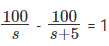(Difference of time take in both the cases is 1 hour)
=> 100s + 500 - 100s = s(s+5)
=> s(s+5) = 500 (One should practice to solve these kind of equations directly by factorizing 500 into 20 X 25, instead of solving the entire quadratic. However, if you are more comfortable with quadratic, keep using it. More important thing is to avoid silly
mistakes once you have got the concept right)
Solving we get, s = 20 Km/hr
Therefore, Increased speed = s + 5 = 25 km/hr
We could have alternatively identified increase in speed by 5 Km/hr as a constant.
Therefore, we could write the equations as: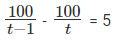Solving, we get t = 5 hrs, t - 1 = 4hrs. Therefore, increased speed =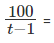25 Km/hr.
The question is " What is the changed speed of Alok?"
Hence, the answer is 25 Km/hr.

Question 22 : Akash when going slower by 15 Km/hr, reaches late by 45 hours. If he goes faster by 10 Km/hr from his original speed, he reaches early by 20 hours than the original time. Find the distance he covers.
A. 8750 Km
B. 9750 Km
C. 1000 Km
D. 3750 Km
Option: B
Explanation:
You may solve for D and S by writing the equations as: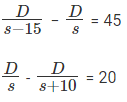You can solve these two equations to get D = 9750 Km which is a perfectly fine approach. However, if you are comfortable with alligation, you may save time on such TSD question as: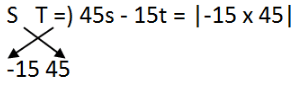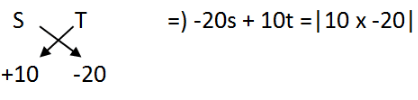Both these equations can be solved quickly to get s = 65 Km/hr, t = 150 hr. Therefore, D = 9750 Km. I want to reiterate that using the first approach is perfectly fine. That's how I would do it. It is better to spend 20 seconds extra and get the right answer instead of getting it wrong with something you are not comfortable with.
The question is "Find the distance he covers."
Hence, he covers 9750 km.

Question 23 : Raj was travelling to his hometown from Mumbai. He met with a small accident 80 Km away from Mumbai and continued the remaining journey at 4/5 of his original speed and reached his hometown 1 hour and 24 minutes late. If he had met with the accident 40 Km further, he would have been an hour late. i) What is Raj's normal speed?
A) 20 Km/hr B) 15 Km/hr C) 30 Km/hr D) 25 Km/hr
ii) What is the distance between Mumbai and Raj's hometown?
A) 140 Km B) 200 Km C) 220 Km D) 250 Km
Option: D and C
Explanation:
Scenario 1: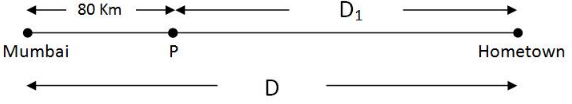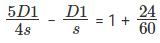(always write minutes in this form if the unit is Km/hr)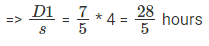Scenario 2: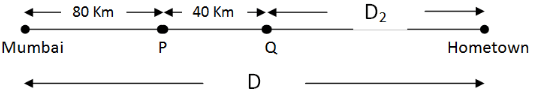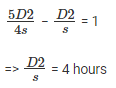i) Therefore, in his normal speed, he can travel 40 Km in 28/5 - 4 = 8/5 hours so,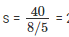25 Km/hr.
ii) D1/s = 28/5 => D1 = 140 Km. Therefore, total distance D = 140 + 80 = 220 Km.
The question is "i) What is Raj's normal speed? and ii) What is the distance between Mumbai and Raj's hometown? "
Hence, the answer is 25 Km/hr and 220 Km.

Question 24: Two persons A and B start moving at each other from point P and Q respectively which are 1400 Km apart. Speed of A is 50 Km/hr and that of B is 20 Km/hr. How far is A from Q when he meets B for the 22nd time?
A. 1000 km
B. 400 km
C. 800 km
D. 1400 km
Option: B
Explanation:
Total distance travelled by both of them for 22nd meeting = 1400 + (21 * 2 * 1400) = 43 * 1400
Distance travelled by each will be in proportion of their speed :
Therefore, distance travelled by A = 50/(50 + 20) * 43 * 1400 = 43000 (Note - Always do
complicated calculations at last because things cancel out generally)
Now, for every odd multiple of 1400, A will be at Q and for every even multiple of 1400 A will be at P. So, at 42000 Km (1400 x 30, even multiple) A will beat P. So at their 22
meeting, A will be 1000 Km from P, therefore, 400 Km from Q.
The question is "How far is A from Q when he meets B for the 22nd time?"
Hence, the answer is 400 km

Question 25: Two persons A and B start moving from point P towards Q which are 1400 Km apart. Speed of A is 50 Km/hr and that of B is 20 Km/hr. How far is A from Q when he meets B for the 22nd time?
A. 1000 Km
B. 600 Km
C. 800 Km
D.1400 Km
Option: B
Explanation:
For 22nd meeting, total distance travelled = 22 * 2 * 1400 Km
Distance travelled by A = 5/7* 44 * 1400 = 44000 Km (1400 * 31 + 600).
Therefore, A would be 600 Km from Q.
The question is " How far is A from Q when he meets B for the 22nd time? "
Hence, the answer is 600 Km

Question 26: Two trains A and B are 100 m and 150 m long and are moving at one another at 54 Km/hr and 36 Km/hr respectively. Arun is sitting on coach B1 of train A. Calculate the time taken by Arun to completely cross Train B.
A. 10 s
B. 6 s
C. 4 s
D. 8 s
Option: B
Explanation:
Speed of A = 54 * 1000/60∗60 = 15 m/s
Speed of B = 36 * 1000/60∗60 = 10 m/s
Relative speed = S1 + S2 = 15 + 10 m/s = 25 m/s
The length that needs to be crossed = length of train B = 150 m.
Therefore time taken = 150/25 = 6s
What is the time taken for trains to completely cross each other? The length that needs to
be crossed = 100 + 150 = 250 m. Time taken = 250/25 = 10 s
The question is " Calculate the time taken by Arun to completely cross Train B. "
Hence, the answer is 6 s.

Question 27 : Two trains left from two stations P and Q towards station Q and station P respectively. 3 hours after they met, they were 675 Km apart. First train arrived at its destination 16 hours after their meeting and the second train arrived at its destination 25 hours after their meeting. How long did it take for the first train to make the whole trip?
A. 18h
B. 36h
C. 25h
D. 48h
Option: B
Explanation: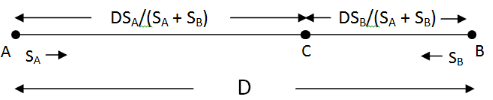Total distance travelled by both the trains before meeting = D. This distance will be covered in the proportion of their speeds. Clarify with the diagram
3 hours after meeting distance travelled by A = SA * 3 and by B = SB * 3
Therefore, 3 (SA + SB) = 675 => SA + SB = 225.
Now the remaining distance to be covered by first train is DSB/(SA + SB)
Therefore, time taken =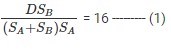Similarly,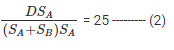Dividing equation 1 by 2 -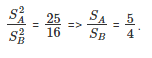Therefore, SA + 4/5 * S= 225
=> SA = 125 Km/hr and SB = 100 Km/hr
From equation 2,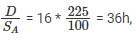which is the time taken for the first train to complete the journey.
The question is "How long did it take for the first train to make the whole trip?"
Hence,it took 36h for the first train to make the whole trip.

Question 28 : Arjun travels from A to B, a distance of 200 Km at the speed of 40 Km/hr. At the same time, Rakesh starts from point C at a speed of 20 Km/hr along a roadwhich is perpendicular to AB. Find the time in which Arjun & Rakesh will be closer to each other?
A. 1.5 hr
B. 3.33 hr
C. 5 hr
D. 4 hr
Option: B
Explanation: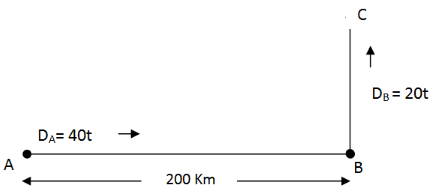Distance between the two at any time t, D = √((200−40t)2+(20t)2)
If X is minimum, then √will also be minimum and vice versa.
So, we need to minimize (200−40t)2+(20t)2
Or, we need to minimize 400 (20 – 2t)2 + 400t2
Or, we need to minimize (20 – 2t)2 + t2
Or, we need to minimize 400 + 4t2 – 80t + t2
Or, we need to minimize 5t2 – 80t + 400
Or, we need to minimize t2 – 16t + 80
Or, we need to minimize t2 – 16t + 64 + 16
Or, we need to minimize (t – 4)2 + 16
Or, t should be 4.
Or, the distance will be minimum in 4 hours’ time.
WE could do this using differentiation also, but we do not need to know differentiation to answer this question
The question is "Find the time in which Arjun & Rakesh will be closer to each
other?"
Hence, the answer is 4 hr.

The document Speed, Time & Distance: Solved Examples- 3 | CSAT Preparation - UPSC is a part of the UPSC Course CSAT Preparation.
All you need of UPSC at this link: UPSC

## CSAT Preparation

197 videos|151 docs|200 tests

## FAQs on Speed, Time & Distance: Solved Examples- 3 - CSAT Preparation - UPSC

 1. What is the formula to calculate speed?Ans. The formula to calculate speed is speed = distance / time.
 2. How is time related to speed and distance?Ans. Time is inversely related to speed and directly related to distance. This means that as speed increases, time decreases, and as distance increases, time also increases.
 3. How can I calculate the distance traveled if I know the speed and time?Ans. To calculate the distance traveled, you can use the formula distance = speed x time. Simply multiply the speed by the time to get the distance.
 4. What units are typically used to measure speed, time, and distance?Ans. Speed is typically measured in units such as kilometers per hour (km/h) or meters per second (m/s). Time is measured in units such as hours, minutes, or seconds. Distance is measured in units such as kilometers or meters.
 5. How can I convert speed from one unit to another?Ans. To convert speed from one unit to another, you can use conversion factors. For example, to convert from kilometers per hour (km/h) to meters per second (m/s), you can multiply the speed in km/h by 1000/3600.

## CSAT Preparation

197 videos|151 docs|200 testsExplore Courses for UPSC exam### How to Prepare for UPSC

Read our guide to prepare for UPSC which is created by Toppers & the best Teachers
Signup to see your scores go up within 7 days! Learn & Practice with 1000+ FREE Notes, Videos & Tests.
10M+ students study on EduRev
Track your progress, build streaks, highlight & save important lessons and more!
Related Searches

,

,

,

,

,

,

,

,

,

,

,

,

,

,

,

,

,

,

,

,

,

,

,

,

;